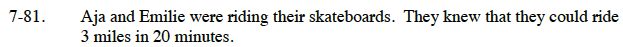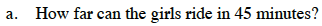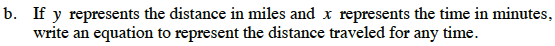### Home > CC3 > Chapter 7 > Lesson 7.2.5 > Problem7-81

7-81.
1. Aja and Emilie were riding their skateboards. They knew that they could ride 3 miles in 20 minutes. Homework Help ✎

1. How far can the girls ride in 45 minutes?

2. If y represents the distance in miles and x represents the time in minutes, write an equation to represent the distance traveled for any time.Set up a proportion.

$\frac{3}{20}=\frac{\it y}{45}$

20y = 135

y = 6.75 milesYou can also use a proportion for this problem.

$\frac{3}{20}=\frac{\it y}{\it x}$

Multiply both sides by x to simplify the equation.